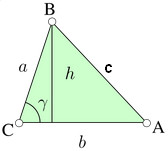## Calculate Perimeter of a TrianglePerimeter = a + b + c

 Enter your values: Length (a): Length (b): Length (c): Results: Perimeter of a Triangle:

A triangle is one of the basic shapes in geometry: a polygon with three corners or vertices and three sides or edges which are line segments.

Thinkcalculator.com provides you helpful and handy calculator resources.